Monday, 2019 May 20, 23:24
Welcome! Guest | Register | Log in

Menu
Форма входа
Search
Our friends
Статистика

Total online: 1
Guests: 1
Users: 0

# Chapter Five.

It is opened a physic law: the new kind of motion allows traveling on spaceships from a star to other.

"Science is improving by experience”

Francis Bacon (1561-1626)

All of the existing space flying machines move due to inertia, gained by inflaming of combustible. On leaving of gas from the turbine the machine gets velocity. On Earth every mobile technique is repulsing from something: earth’s surface, water, air. For example, when the car is towing, it can’t move from place, because the traction upon ground is absent. The helicopter, to fly upwards, pushes the air downwards. The ships push the water backwards to move onward. If follow the Law of conservation of momentum, then results that nothing and no one can move till it doesn’t get a push from outside or it doesn’t repulse form something. The made experiments prove the new regularity: it is possible to get a push by not repulsing from anything. The given affirmation goes in contradiction with the generally accepted Law of conservation of momentum. On the basis of the new law it is possible to construct spaceships, which will be fed not with ordinary combustible, and will fundamentally move in a new way. For realization of construction of such flying machines correct calculations are necessary, which should be taken in accordance with the given physical phenomenon.

There exists an opinion: physics is a fundamental science, all of the laws are proved and stated in physical textbooks, you can’t doubt, correct or complement them. Persons appear, which at some moment realize the fact of insufficiency of our knowledge in any part of science. They discover the "blank spaces”, trying to explain that what wasn’t explained before.

For instance, science can’t give an answer, why the bullet, in comparison with the gun, gets so more energy from enflaming of gunpowder in stem. The scientists consider that this physical phenomenon is subordinated to Law of momentum, but it is not subordinated to the Law of conservation of energy. It is discovered the regularity, which gives explanation to this physical phenomenon.
Due to that we have included time in the formula of the Law of conservation of momentum, we could calculate that what before wasn’t being possible to calculate by physical laws.
If examine the phenomenon with the gun, we observe the following: the person, which shot from a gun, felt only a push in the shoulder, and the person, which had been shot, got a strong hit or he was heavily rejected away. According to the Law of conservation of momentum when shooting both should be hit or rejected equally, because they aren’t influenced by any power from outside.
It is proposed a concrete way, which solves the problem and explains this phenomenon.
By means of the device it is possible to demonstrate in practice why two different masses gain different momentums from the action of one and same power. This invention makes possible the motivation of the new physical law.
We propose a device, by means of which it is possible to make several experiments for measurement of kinetic energy of objects – some balls with different masses and it can be used in physical laboratories for a detailed explanation of physic laws (for instance, the phenomenon with the gun).
The essence of the invention consists in uncovering of meaning of the Law of momentum. After all, the physical laws are discovered for people to get a fuller notion about the nature.
This invention pursues the goal to demonstrate on practice by means of device the physical phenomenon, which gives the explaining why the bullet, in comparison with the gun, gets so much energy from enflaming of gunpowder in stem.

How does the given device look?

The device (figure-1) is composed of corpus 1. Inside of corpus 1 are installed spring 2 and spring 3, the thread 4 is tied to them, near spring 2 it is found hanging ball 5, near the spring 3 it is hanging the ball 6, and in the lower part of corpus it is situated the pushcart 7.
We are making the first experiment: the spring 2 and the spring 3, equal by resistances and sizes, are compressed by the thread 4. Near the spring 2 is found ball 5 (mass – 200 g), and near the spring 3 is the ball 6 (mass – 100 g).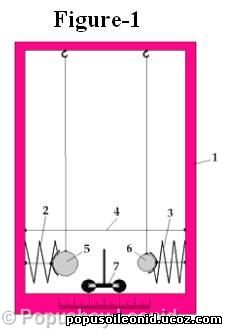When the thread 4 is cut, the spring 2 and the spring 3 unclench. The spring 2 and the spring 3 when unclenching pushes ball 5 and ball 6, as a result both of the balls get kinetic energy and hit the pushcart 7. We see that ball 6 with mass of 100 g hit first the pushcart 7 (see figure-2). The ball with the smaller mass gains velocity in a shorter time period, than the heavier ball does.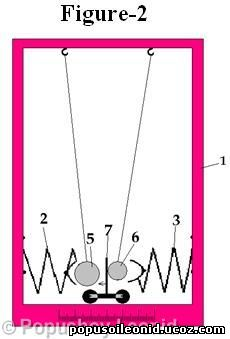When we are making the first experiment, we know that the potential energies of springs 2 and 3 are equal, because their sizes are identical and they are compressed equally.
It is different just the time of transmission of energy to balls. We know that the small ball with mass of 100 g hit the pushcart 7 first, this proves that the spring 3 transmitted quicker its energy to the small ball. It follows that the physical bodies with different masses gain equal kinetic energy in different time periods from one and same power.

m1>m2 – (m1 – ball 5 with mass of 200 g, m2 – ball 6 with mass of 100 g);
tm1>tm2 – (the time of transmission of energy to balls from springs);
Wkm1=Wkm2 – (the kinetic energy of balls).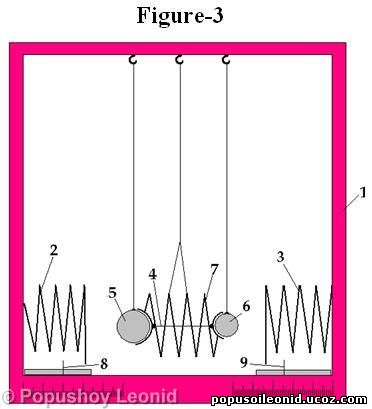Hereinafter we change the construction of device, as follows, we add the third spring. The device (figure-3) consists of corpus 1, inside of which are installed spring 2 and spring 3, is hung ball 5, spring 7 and ball 6, and in the lower part of corpus 1 are found indicators 8 and 9. Thread 4 fixes the spring 7 in a compressed condition. By means of this device we make the second experiment: the spring 7 is compressed by thread 4, on the left and on the right are situated two balls 5 and 6 with different masses. The ball 5 is having a mass of 200 g, and the ball 6 has a mass of 100 g. When cutting the thread 4, the spring 7 is straightening and pushes balls
5 and 6 in opposite sides.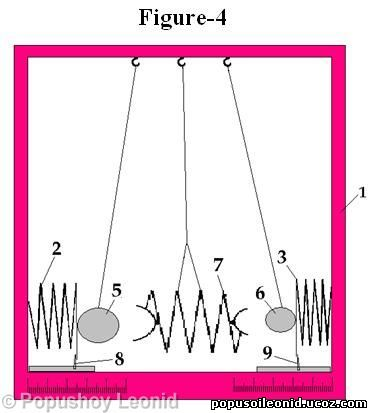Ball 5 when hitting the spring 2 compresses it and the ball 6 when hitting the spring 3 also compresses it. The springs 2 and 3 are equal in resistances and sizes. By means of indicators 8 and 9 we notice the kinetic energy of balls 5 and 6, which got a push from spring 7 (see figure-4, which demonstrates the experiment in dynamic).
After making the second experiment we see that ball 6 with mass of 100 g compresses stronger the spring than ball 5 with mass of 200 g. This happens because the object with a smaller mass (ball 6 – 100 g) gains kinetic energy from spring 7 quicker than the object with a greater mass (ball 5 – 200 g). We reach the conclusion: the object with the smaller mass needs a shorter time period to gain kinetic energy. The spring 7 is found between balls 5 and 6, when straightening it transmits potential energy to balls in one and same time. In such case the ball with a smaller mass in the same time period gains more kinetic energy.
It follows that physical bodies with different masses gain kinetic energy of different values in an equal time period by the influence of one and same power.

m1>m2 – (mass of ball 1 is greater than mass of ball 2);
tm1=tm2 – (the equal time periods of energy transmitting to bath of balls from spring);
wkm1<wkm2 – (kinetic energy of ball 1 is smaller than the kinetic energy of ball 2).

After making these two experiments, it is coming to light the contradiction between theory and practice: the essence of the third Newton’s law doesn’t correspond to the made experiments. The third Newton’s law says: "To every action there is always opposed an equal reaction: or the mutual actions of two bodies upon each other are always equal, and directed to contrary parts.”, and more briefly:"To every action there is an equal and opposite reaction”. The discrepancy between this law and the practice is concluded in that the power of action is not equal to the power of the opposite reaction. The first experiment proves that from one and same power two different objects get equal momentums, but different kinetic energies. The second experiment proves that from one and same power two different objects get equal momentums, but different kinetic energies.
The third Newton’s law is about the equality of powers, in fact, the indicated equality is absent: when the momentums are equal, the energies are different, and if the energies are equal, then the momentums are different.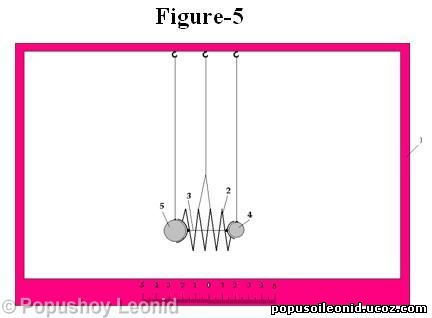For more clarity we demonstrate this phenomenon with the help of figure-5.
The spring 2 is compressed with thread 3.
When cutting the thread 3, spring 2 pushes balls 4 and 5 in opposite directions. Figure-6 demonstrates the experiment in dynamic.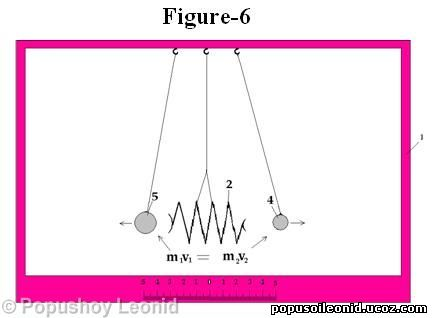If being guided by the Law of conservation of momentum, it turns that the pulled balls 4 and 5 should get an equal momentum for the centre of system to be saved. For instance: ball 4 with mass of 100 g got a velocity of 10 m/s (m2v2), and the ball 5 with mass of 200 g gained a velocity of 5 m/s (m1v1). Balls 4 and 5 get an equal momentum from the spring 2: m1v1=m2v2

If follow the formula of kinetic energy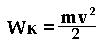then ball 4 with mass of 100 g gets kinetic energy from spring 2 (Figure-6).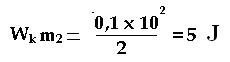And the ball 5 with mass of 200 g gets from the same spring kinetic energy twice smaller (Figure-6).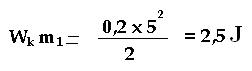For example, the spring 2 in a compressed condition had a potential energy of 7.5 J. After its straightening, if excluding the loss, ball 4 got 5 J, and ball 5 gained 2.5 J. The ball 4 with mass of 100 g got kinetic energy twice bigger than the ball 5 with mass of 200 g.
Figure-7 demonstrates that when balls are hitting the springs, these are compressed differently and, respectively, the energy of springs is also different.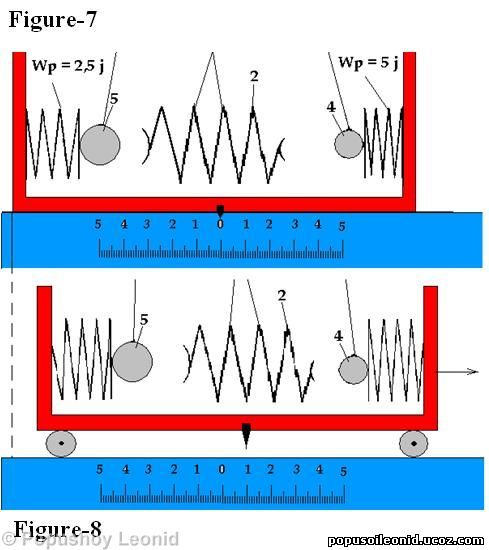The sense of this phenomenon becomes clear, if comparing figure-7 and figure-8. In figure-7 we see: balls 4 and 5 when gaining equal momentums have different kinetic energies and in an equal time period compress the springs differently, because the pushcart is standing still. If examining the figure-8, where the pushcart is easily moving, both of the springs, which are fixed to pushcart, when compressing try to equalize their resistances, and provoke the moving of pushcart. The figure-8 demonstrates that the pushcart is being displaced in the direction of the stronger hit, struck from the lighter ball. Though the balls have equal momentum, but because of they possess different kinetic energies and execute different works, the centre of system changes it position. The pushcart is founding in a closed system, since no power influences from outside.
The given experiment helps to understand the phenomenon about the gun: why in the moment when shooting the bullet gains more kinetic energy in comparison with the gun? The Kalashnikov gun’s bullet when getting out from the stem has kinetic energy of 2020 J and the gun itself has the kinetic energy of 5 J. The difference of these two energies is equal to 2015 J.
See figure-9.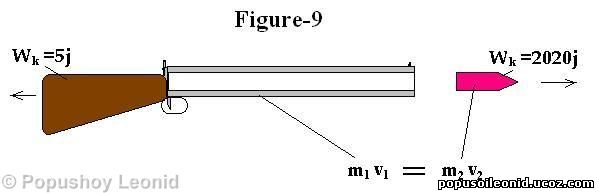m1v1 (momentum of the bullet) = kinetic energy of 2020 J;
m2v2 (momentum of the gun) = kinetic energy of 5 J.

The bullet and the gun gain equal momentum m1v1= m2v2, hereinafter both of masses are having different kinetic energies and can execute different works, as a result, when clashing with other objects, the centre of the system changes. The bullet with its kinetic energy, when clashing with an object, will throw it farther aside than the gun. The person, which shot from gun, and the one in whom has being shot, are found in a closed system, since no power is influencing from the outside. Due to the energy, formed in the gun, one was struck heavily, and the second easier. If being guided by the Law of conservation of momentum, both should be struck equally for either both of them to be displaced equally from the centre of the system.

The bullet’s energy and gun’s energy are directed in opposite sides and numerically they are not equal. The physical phenomenon, which is having place when shooting, proves that the equality is not being saved.
On the basis of the third Newton’s law it is not possible to explain the phenomenon with gun.
As a result of the made experiments it is becoming obviously that the Law of conservation of momentum acts till the objects, which got equal momentum, don’t clash with other objects. The Law confirms that the interaction of bodies, which form a closed system, brings only to the changing of momentums between these bodies, but it can’t change the motion of the system as a whole: when any interaction between bodies, which form a closed system, the velocity of the motion of inertia’s centre doesn’t change.
But the experiment proves the opposite: the centre of the system does change.

The conclusion of the fifth chapter is the seventh regularity:
If including the time (t) in the Law of conservation of momentum, then it helps to understand, why different objects with the same momentums have different kinetic energies, and, also gives the explanation to the phenomenon with gun.

1   2   3   4   5   6